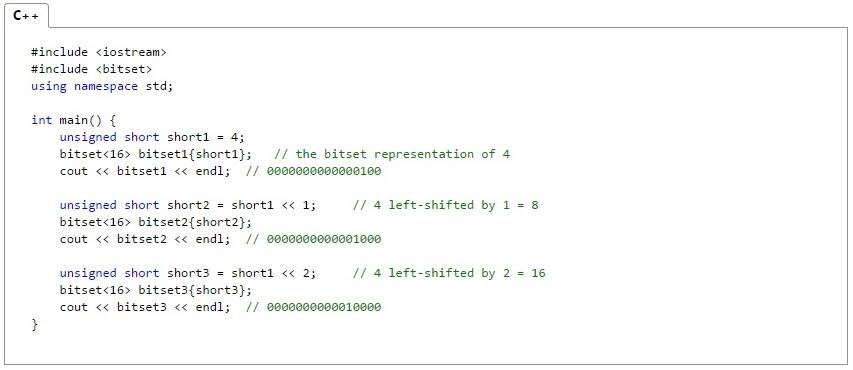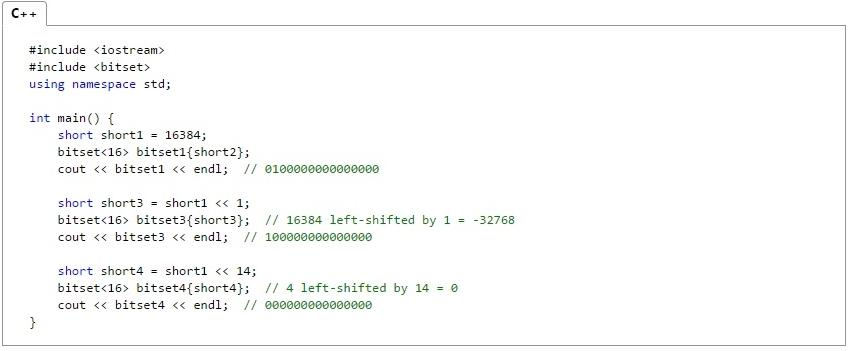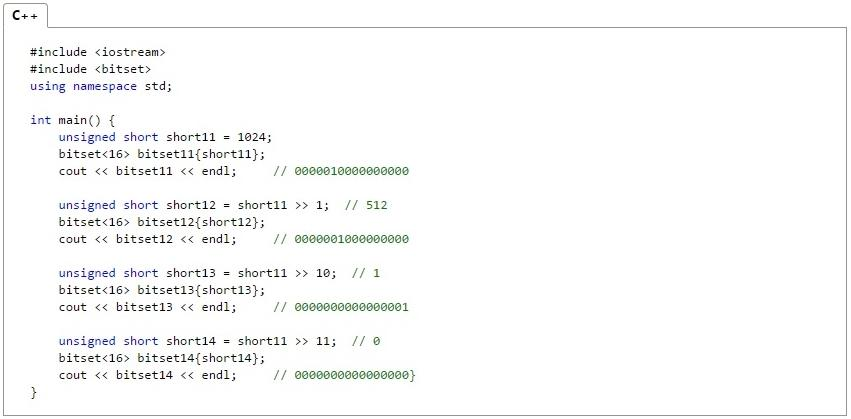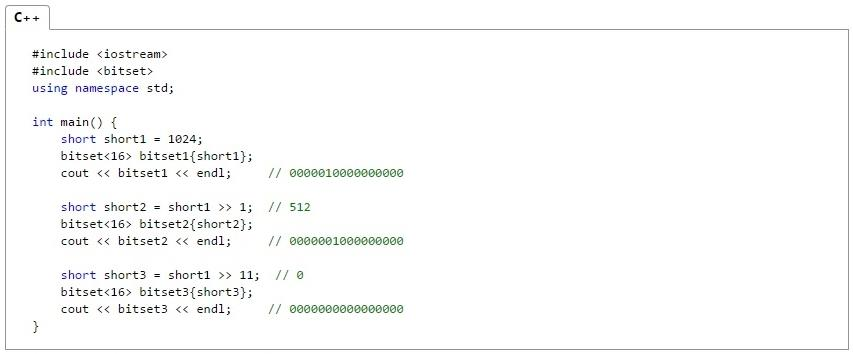# Bit Shift and Bit Manipulation

### Introduction

The computer programs are based on essentially the realization of numerous mathematical and arithmetical operations of different complexities. This article shows the functioning of those operations in the language C++ program.
The key operator that will be analyzed in this text is bit manipulation. Why has the importance of addressing the operating concepts and understand the basic principles of bit manipulation operators to reach the object of study, which is the bit-shift operation.

### Bit manipulation operators

Handling or treatment operators (bitwise) perform logical operations on each of the bits of the operands. These operations are comparable in efficiency and speed to their equivalents in fitting language.
Each bit manipulation operator performs a bitwise logical operation on internal data. Bit manipulation operators apply only to variables and constants char, intand long, and not floating point data. If we consider that the binary numbers are set to 1 and 0 (in certain bits), these 1 and 0 if you manipulate to produce the results for each of the operators.
Below we can see the truth tables that describe the actions that perform various operators on the various bit patterns of given int (char or long).

 Operator Operation & Y (And bitwise logic) || The (OR) logical bitwise (inclusive) ^ (XOR) logic (exclusive) bitwise (OR exclusive, XOR) ˜ A complement (inversion of all the bits) << Bitshiftleft >> Bit shift right

A&B == C
0&0 == 0
0&1 == 0
1&0 == 0
1&1 == 1
A|| B == C
0 || 0 == 0
0 || 1 == 1
1 || 0 == 1
1 || 1 == 1
A ^ B == C
0 ^ 0 == 0
0 ^ 1 == 1
1 ^ 0 == 1
1 ^ 1 == 0
A ~ A
1 0
0 1

Another type of operators available for bit manipulation, are abbreviated assignment operators. These operators are shown in the table below:
 Symbol Use Description <<= The << = b Shifts to the left b bits and assign the result >>= The >> = b Shifts to the right b bits and assign the result &= a & = b Assings the a the value to & (b). ˆ= a ˆ= b Establishes a the a ^ b |= the | (b) Establishes the a | b

#### Bit shift operators (>>,<<)

The analysis of the bit-shift operators is the main point of this article.

Bit-shift operators originates along with the C language, which was invented and implemented primarily by Dennis Ritchie in a DEC PDP-11 using the UNIX operating system. The C language is the result of a development process that began with an older language, called BCPL, which is still in use in its original form in Europe. BCPL was developed by Martin Richards and influenced a language called B, invented by Ken Thompson. In early 1970, B has led to the development of C.

For many years, in fact, the default for C was the version that ships with the operating system version 5 UNIS. He is described in The C Programming Language, by Brian Kernighan and Dennis Ritchie (Englewood Cliffs, N.J.: Prentice Hall, 1978). With the popularity of computers, a large number of implementations of C was created. Almost by miracle, the accepted sources for these implementations were highly compatible. (That is, a program written with one of them could normally be successfully compiled using another). However, there is no default, there were discrepancies. To remedy this situation, the American National Standards Institute (ANSI) has established, in the summer of 1983, a Committee to create a standard that would define once and for all the language c. ANSI standard Committee was wrapping up the formal process of adoption. All major C compilers have already implemented the ANSI C standard.

##### How does the shift operators
The bitwise shift operators (>>, <<) move all the bits of a variable to the right or to the left. The General form of the right shift command is:

variable >> number of bit positions

The General form of the left offset command is:

variable << number of bit positions

As the bits are shifted to one end, zeros are placed in another. Is valid remember that an offset is not a rotation. That is, the bits that go out by one end won't be back for another. The displaced people are lost and zeros are placed.

Bit-shift operations can be useful when it decodes the input of an external device, such as a D/A converter, and when you read state information. The shift operators on bit level can also multiply and divide integers quickly. An offset to the right effectively multiplies a number by 2 and an offset to the left is divided by 2, as shown in the table below.

 Unsigned char x x every sentence Value of x x = 7 00000111 7 x = x 1 << 00001110 14 x = x 3 << 01110000 112 x = x 2 << 11000000 192 x = x 1 >> 01100000 96 x = x 2 >> 00011000 24

Each offset to the left multiplies by 2. Note that information is lost after the x << 2 because a bit was moved out.

Each offset to the right divides by 2. Therefore, the subsequent divisions do not bring back bits previously lost.

##### Offsets to the left
The left-shift operator causes the bits in the shift-expression are shifted to the left by the number of positions specified by the additive-expression. The positions of the bits were released by displacement are filled with zeros. An offset to the left is a logical shift. That is, the bits that are shifted from the end are eliminated, including the sign bit.

The example below shows left shift operations using unsigned numbers.The following example shows what happens in visual C++ when 1 bit is shifted to left on its sign bit:##### Offsets to the right
The right-shift operator causes the bits in the pattern shift-expression is shifted to the right by the number of positions specified by the additive-expression. For unsigned numbers, bit positions that were released by displacement are filled with zeros. For signed numbers, the sign bit is used to fill the vacant bit positions. That is, if the number is positive, 0 will be used, and if the number is negative, 1 will be used.

The example below shows right shift operations using unsigned numbers:The next example shows right shift operations with positive numbers with sign:Bit-shift operators are equivalent to SHR instruction (>>) and SHL (<<) 80 x 86 microprocessors. As was shown in this article, the basic principle of operation is to make the offset of n positions of the bits of the operand, where n is an integer. The number of bits shifted depends on the value to the right of the operator.